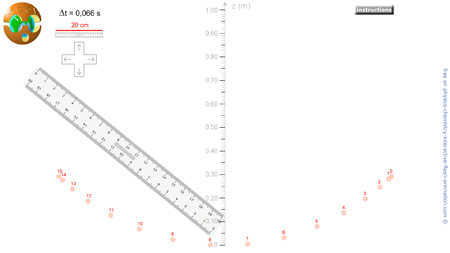SIMPLE GRAVITY PENDULUM

# EXPERIMENTAL ACTIVITY

Description: Interactive flash animation to simulate the measurement of speed in pendulum case: mesuring also the height of the ball, it's possible to know the kinetic energy and potential one.

Instructions:

The ball weighed: m = 100 g. Use g = 9,8 N.kg-1.

a. We compare the ball to a point-like object. Give the expression of its kinetic energy.

b. When using the chrono or a time interval marker (Dt constant), how do you asses the speed of the object, for example A4 ?

c. Calculate Ek for each recorded position (except the two outliers). Record your results in a table.

d. Draw the graph of the evolution of Ek vs time.

a. Give the expression of the gravitational potential energy.

b. Calculate Ep for each recorded position (except the two extremes). Record your results in the table.

c. Draw the graph of the evolution of Ep vs time.

a. Plot the graph of E = Ek + Ep vs time.

b. Comment on the evolution of E.

a. Establish an inventory of the forces acting on the ball. What can be said about the work of forces other than weight ?

b. Draw a diagram of the energy exchange between the starting position and a position during the movement. Explain the evolution of E.

c. Were the results of this measurement applicable to a large number of the oscillations ? Justifiy.

Definition:

Simple pendulum: Hypothetical pendulum suspended by a massless and frictionless thread of constant length.

Video: PCCL does not post a video here as it is up to the students to complete the activity.